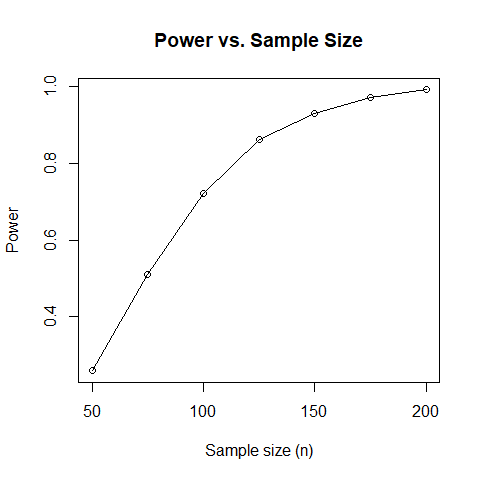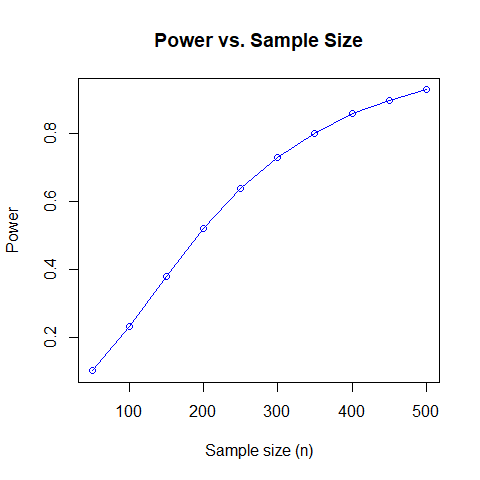Using the smartsizer Package

2021-01-04

library(smartsizer)

Introduction

The $$\texttt{smartsizer}$$ package is designed to assist investigators who are planning sequential, multiple assignment, randomized trials (SMART) in determining the sample size of individuals to enroll. The main goal motivating SMART designs is determination of optimal dynamic treatment regime (DTR). Therefore, investigators are interested in sizing SMARTs based on the ability to screen out DTRs inferior to the best by a specified amount $$\Delta_{\mathrm{min}}$$ with probability $$1-\beta$$ while identifying the true best with a specified probability $$1-\alpha$$. The $$\texttt{smartsizer}$$ package utilizes the Multiple Comparisons with the Best (MCB) methodology for determining a set of best DTRs while adjusting for multiple comparisons.

Using $$\texttt{smartsizer}$$, investigators may perform power analyses for an arbitrary type of SMART.

Details on the methodology and guidelines for choosing the covariance matrix are available in ‘’W.J. Artman, I. Nahum-Shani, T. Wu, J.R. Mckay, and A. Ertefaie. Power analysis in a SMART design: sample size estimation for determining the best embedded dynamic treatment regime. Biostatistics, 2018’’.

Power Calculation

The $$\texttt{computePower}$$ function computes the power as a function of the given covariance matrix, effect sizes, type I error rate, and sample size. The power is the probability of excluding from the set of best all embedded DTR (EDTR) which are inferior to the true best DTR by $$\Delta_{\mathrm{min}}$$ or more.

Power Function Arguments

The arguments of the $$\texttt{computePower}$$ function are as follows:
1. $$\texttt{V}$$: the covariance matrix of mean EDTR outcome estimators.
2. $$\texttt{Delta}$$: the vector of effect sizes.
3. $$\texttt{min_Delta}$$: the minimum detectable effect size.
4. $$\texttt{alpha}$$: the type I error rate (less than 0.5 and greater than 0).
5. $$\texttt{sample_size}$$: the number of individuals enrolled in the SMART.

Power Calculation Examples

We will now look at how to use the computePower function. Consider the following covariance matrices:

V1 <- diag(6)
V2 <- rbind(c(1, 0.2, 0, 0), c(0.2, 1, 0, 0), c(0 , 0, 1, 0.2), c(0, 0, 0.2, 1))

$$\texttt{V1}$$ corresponds to a SMART in which there are six EDTRs. $$\texttt{V2}$$ corresponds to a SMART in which there are four EDTRs.

Let the vector of effect sizes be

Delta1 <- c(0, 0.5, 0.5, 0.5, 0.5, 0.5)
min_Delta1 <- 0.5

Delta2 <- c(0, 0.2, 0.3, 1.5)
min_Delta2 <- 0.2

In both Delta1 and Delta2, we are assuming the first DTR is best. For Delta1 we are being as conservative as possible by assuming all other DTRs are the minimum detectable effect size away. In the first example, the power is the probability of excluding all EDTRs 0.5 away from the first EDTR or more. In particular, the power is the probability of excluding $$\mathrm{EDTR}_2,...,\mathrm{EDTR}_6$$.

In the second example, we have information that some of the effect sizes are further away from the best than than the min detectable effect size which yields greater power. The power is the probability of excluding $$\mathrm{EDTR}_2,\mathrm{EDTR}_3,\mathrm{EDTR}_4$$ from the set of best.

We assume the type I error rate to be at most $$0.05$$ so that the best DTR is included in the set of best with probability at least $$1-\alpha$$. The power is computed as follows:

computePower(V1, Delta1, min_Delta1, alpha = 0.05, sample_size = 120)
#>  0.83
computePower(V2, Delta2, min_Delta2, alpha = 0.05, sample_size = 250)
#>  0.64

We see that the power is 83% in the first example and 64% in the second.

Sample Size Estimation

The $$\texttt{computeSampleSize}$$ function estimates the minimum number of individuals that need to be enrolled in order to achieve a specified power.

Sample Size Function Arguments

The arguments of the computeSampleSize function are as follows:
1. $$\texttt{V}$$: the covariance matrix of mean EDTR outcome estimators.
2. $$\texttt{Delta}$$: the vector of effect sizes.
3. $$\texttt{min_Delta}$$: the minimum detectable effect size.
4. $$\texttt{alpha}$$: the type I error rate (less than 0.5 and greater than 0).
5. $$\texttt{power}$$: the desired power.

Sample Size Estimation Examples

We will look at the same example as in the power calculation. Let

V1 <- diag(6)
V2 <- rbind(c(1, 0.2, 0, 0), c(0.2, 1, 0, 0), c(0 , 0, 1, 0.2), c(0, 0, 0.2, 1))

Assume the vector of effect sizes are

Delta1 <- c(0, 0.5, 0.5, 0.5, 0.5, 0.5)
min_Delta1 <- 0.5

Delta2 <- c(0, 0.2, 0.3, 1.5)
min_Delta2 <- 0.2
computeSampleSize(V1, Delta1, min_Delta1, alpha = 0.05, desired_power = 0.8)
#>  114
computeSampleSize(V2, Delta2, min_Delta2, alpha = 0.05, desired_power = 0.8)
#>  346

The sample size in the first example is the minimum number of individuals which need to be enrolled in order achieve 80% power of excluding $$\mathrm{EDTR}_2,\mathrm{EDTR}_3,\mathrm{EDTR}_4,\mathrm{EDTR}_5, \text{ and }\mathrm{EDTR}_6$$ from the set of best.

The second example computes the minimum number of individuals which need to be enrolled in order to achieve 80% power to exclude $$\mathrm{EDTR}_2,\mathrm{EDTR}_3, \text{ and }\mathrm{EDTR}_4$$.

Consequently, 114 individuals need to be enrolled in the first example and 347 individuals need to be enrolled in the second example in order to achieve 80% power.

Computing the Power Over a Grid of Sample Sizes

We now demonstrate the $$\texttt{computePowerBySampleSize}$$ and $$\texttt{plotPowerByN}$$ functions.

$$\texttt{computePowerBySampleSize}$$ computes the power over a grid of sample size values.

computePowerBySampleSize(V1, Delta1, min_Delta1, alpha = 0.05, sample_size_grid = seq(50, 200, 25))
#>  0.26 0.51 0.72 0.86 0.93 0.97 0.99
computePowerBySampleSize(V2, Delta2, min_Delta2, alpha = 0.05, sample_size_grid = seq(50, 500, 50))
#>   0.10 0.23 0.38 0.52 0.64 0.73 0.81 0.86 0.90 0.93

Creating Power Plots

The $$\texttt{plotPowerByN}$$ function plots the power vs. the sample size over a grid of sample size values. The following two example plots demonstrate the function. The power is computed using common random variables to reduce the variance and to speed up generation of the plots.

plotPowerByN(V1, Delta1, min_Delta1, alpha = 0.05, sample_size_grid = seq(50, 200, 25), color = "black")plotPowerByN(V2, Delta2, min_Delta2, alpha = 0.05, sample_size_grid = seq(50, 500, 50), color = "blue")Acknowledgements

Special thanks to Tingting Zhan for discovering a bug in $$\texttt{computePowerBySampleSize}$$.